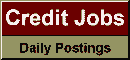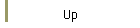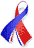DefaultRisk.com the web's biggest credit risk modeling resource.doi> search: A or B Cited by these papersRelated articlesAlternative sources RePEc Posting Export citation to:- HTML- Text (plain)- BibTeX- RIS- ReDIF

On Multivariate Extensions of Value-at-Risk

by Areski Cousin of Université Lyon 1, and
Elena Di Bernardino of CNAM

April 4, 2013

Abstract: In this paper, we introduce two alternative extensions of the classical univariate Value-at-Risk (VaR) in a multivariate setting. The two proposed multivariate VaR are vector-valued measures with the same dimension as the underlying risk portfolio. The lower-orthant VaR is constructed from level sets of multivariate distribution functions whereas the upper-orthant VaR is constructed from level sets of multivariate survival functions. Several properties have been derived. In particular, we show that these risk measures both satisfy the positive homogeneity and the translation invariance property. Comparison between univariate risk measures and components of multivariate VaR are provided. We also analyze how these measures are impacted by a change in marginal distributions, by a change in dependence structure and by a change in risk level. Illustrations are given in the class of Archimedean copulas.

Keywords: Multivariate risk measures, Level sets of distribution functions, Multivariate probability integral transformation, Stochastic orders, Copulas and dependence.

Books Referenced in this paper:  (what is this?)